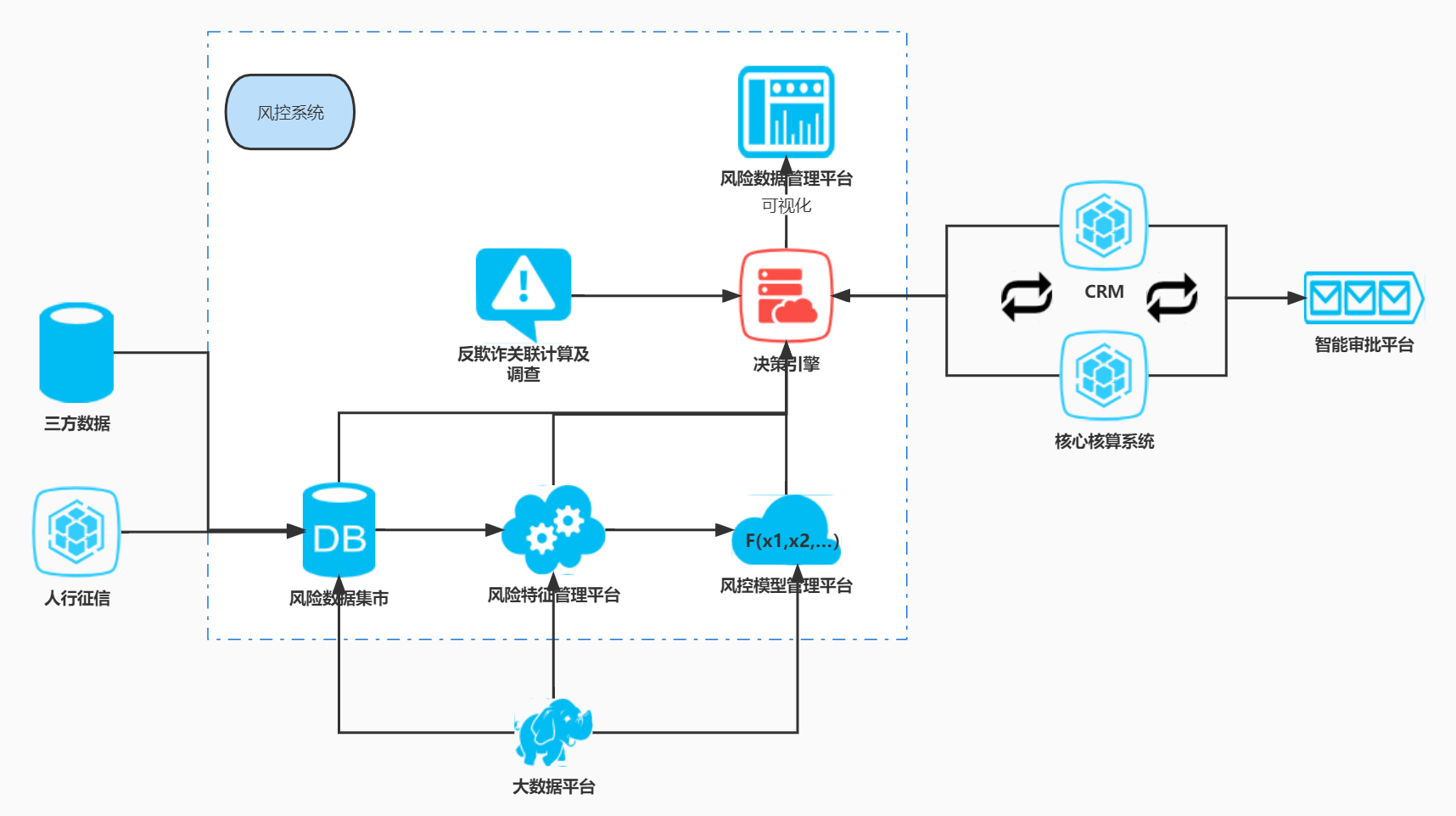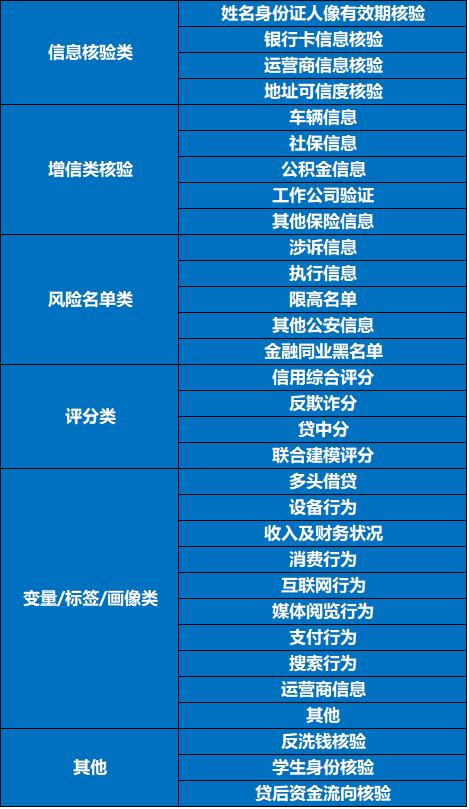0 评论 1731 浏览 1 收藏 27 分钟## 一、《征信业务管理办法》相关

2022年后的三方数据管理，是绕不开此办法的出台及执行的。此管理办法共计八章53条，共计4800字左右，预计十五分钟左右可以阅读完。其也是基于原征信业管理办法、之前试点八家征信机构的实际，所出台的。网上有诸多解读，不再赘述，挑选几个我个人觉得比较重要的点摘录解读。

## 二、三方数据扫盲### 2. 市场上都有什么菜（1）三方数据公司的选择

（2）菜的选择

（3）数据的测试

（4）过会、采购、合同签署

（5）日常对账、开票、付款等

## 三、什么是好的三方数据

### 3. 评估

（1）覆盖率

（2）黑名单准确度

（3）KS

KS(Kolmogorov-Smirnov)：KS用于模型风险区分能力进行评估， 指标衡量的是好坏样本累计分部之间的差值。

（4）WOE/IV

WOE的全称是“WeightofEvidence”，即证据权重。WOE是对原始自变量的一种编码形式。分箱后做一些处理。

pyi是这个组中响应客户（风险模型中，对应的是违约客户，总之，指的是模型中预测变量取值为“是”或者说1的个体）占所有样本中所有响应客户的比例，pni是这个组中未响应客户占样本中所有未响应客户的比例，#yi是这个组中响应客户的数量，#ni是这个组中未响应客户的数量，#yT是样本中所有响应客户的数量，#nT是样本中所有未响应客户的数量。

（5）PSI

（6）贡献度等

— coding: utf-8 —
import numpy as np
import pandas as pd
data_test.shape
data_test.info()
eda=data_test.describe()
path=”
bin=分箱数

#no1.缺失及覆盖率
miss_rate=data_test.isnull().sum()
miss_rate=miss_rate.reset_index()
miss_rate=miss_rate.rename(columns={‘index’:’varname’,0:’miss_num’})
miss_rate[‘miss_rate’]=miss_rate[‘miss_num’]/样本量
miss_rate.to_excel(path)

#no2.黑名单标签类的准确性
verify_true = data_test[((data_test[‘blackflag’]==1)&(data_test[”]==1)) | ((data_test[‘blackflag’]==0)&(data_test[”]==1))]
print(verify_true.shape)

#no3.整体相关性
df_new=data_test.drop([‘cust_name’,’id_no’,’mobile’,’blackflag’],axis=1)
corr=df_new.corr()
corr.to_excel(“path”)

#no4.IV
def cal_iv(data,cut_num,feature,target):
data_cut=pd.qcut(data[feature],cut_num,duplicates=’drop’)
cut_group_all=data[target].groupby(data_cut).count()
cut_y=data[target].groupby(data_cut).sum()
cut_n=cut_group_all-cut_y
df=pd.DataFrame()
df[‘sum’]=cut_group_all
df[‘good_count’]=cut_n
df[‘good_rate’]=df[‘good_count’]/df[‘good_count’].sum()
df.replace({np.inf:0,-np.inf:0},inplace = True)
iv=df[‘iv’].sum()
print(feature,’IV’,iv)
df.to_excel(“path/{}.xlsx”.format(feature))
return(feature,iv)
column_list=df_new.columns.tolist()
df_iv_result=[]
for i in column_list:
df_iv_result.append(cal_iv(data_test,bin,i,’blackflag’))
data_iv=pd.DataFrame(df_iv_result)
data_iv=data_iv.rename(columns={0:’feature’,1:’iv’})
data_iv.to_excel(“path”)

#no5.KS
def cal_ks(data,cut_num,feature,target):
data_cut=pd.cut(data[feature],cut_num)
cut_group_all=data[target].groupby(data_cut).count()
cut_y=data[target].groupby(data_cut).sum()
cut_n=cut_group_all-cut_y
df=pd.DataFrame()
df[‘sum’]=cut_group_all
df[‘good_count’]=cut_n
df[‘good_rate’]=df[‘good_count’]/df[‘good_count’].sum()
df[‘good_rate_sum’]=df[‘good_rate’].cumsum()
ks=df[‘ks’].max()
print(feature,’KS:’,ks)
df.to_excel(“path/{}.xlsx”.format(feature))
return(feature,ks)
df_ks_result=[]
for i in column_list:
a = list(cal_ks(data_test,bin,i,’blackflag’))
df_ks_result.append(a)
data_ks=pd.DataFrame(df_ks_result)
data_ks=data_ks.rename(columns={0:’feature’,1:’ks’})
data_ks.to_excel(“path”)

## 四、结语

1. 目前还没评论，等你发挥！